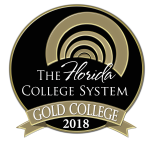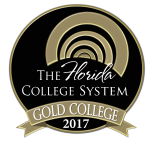MAC2313

## Calculus and Analytic Geometry III

Course Code: MAC2313

Credit Hours: 4

Effective beginning: 2021-22

Course Description:
This course includes the study of lines, planes and surfaces in space; functions of several variables; partial derivatives of functions of several variables; extrema of functions of two variables; iterated integrals using rectangular, polar, cylindrical, and spherical coordinates; differentiation, integration, and applications of vector-valued functions; vector fields and calculations of line integrals and flux integrals, the use of Green’s Theorem, Divergence Theorem, and Stoke’s Theorem. A “C” grade or higher must be earned to advance to a higher-level mathematics course or to satisfy part of the general education requirement in mathematics.

### Course Details

Prerequisites:

A “C” grade or higher in MAC 2312

TBD

##### Required textbooks/ course materials:

Calculus, eleventh Edition, by Larson & Edwards, Brooks/Cole, Cengage Learning

Enhanced WebAssign Printed Access Card for Calculus, Multi Term Courses.  ISBN: 9781337652650

OR

Text + Enhanced WebAssign Homework and eBook LOE Printed Access Card for Multi Term Math and Science.  ISBN: 9781337604741

##### Assignment/course outline:

See your Instructor First Day Handout for individual instructor assignment schedule.

##### Discipline-level learning outcomes:

Area 3 -Math:   Demonstrate Basic Mathematical Skills and Knowledge

The purpose of the mathematics component of the core curriculum is to develop quantitative literacy with an ability to apply basic mathematical tools in the solution of real-world problems.

M-1 Solve mathematical problems by using arithmetic, algebraic, or geometric skills.

M-2 Translate basic mathematical information verbally, numerically, graphically, or symbolically.

M-3 Solve mathematical problems using appropriate technology.

M-4 Interpret mathematical models such as formulas, graphs, tables, or schematics.

M-5 Solve contextual problems using mathematical processes.

SEE CORRELATION FOR TEACHER EDUCATION PROGRAM IN INSTRUCTOR'S FIRST-DAY HANDOUT.

##### Course-level learning outcomes:

Course-level student learning outcomesDiscipline-level learning outcomesAssessment methods

Identify properties of curves and common surfaces in space

Determine derivatives and integrals of vector-valued functions

Solve application problems involving vector-valued functions

Determine the continuity of multivariable functions

Calculate limits of multivariable functions

Find partial derivatives, directional derivatives, and gradients of multivariable functions

Solve optimization problems involving multivariable functions

Solve application problems using the chain rule

Evaluate iterated integrals using rectangular, polar, cylindrical, and spherical coordinates

Examine the properties of vector fields

Evaluate line integrals and flux integrals

M1, M-2, M-4

M-1, M-2, M-4

M-1, M-2, M-3, M-4, M5

M-1, M-4

M-1, M-2, M-3

M-1, M-2, M3, M-4

M-1, M-2, M-3, M-4, M-5

M-1, M-2, M-3, M-5

M-1, M-2, M-4

M-2, M-4

M-1, M-2, M-4, M-5

Unit Tests, Cumulative Final, Homework

##### Means of accomplishing learning outcomes:

Teacher Facilitated: The teacher will be leading class discussions on the material contained in the text during each class period.

Student-centered: The students will take notes and practice solving problems during each class period.

Office Hours: The instructor will be available during office hours for individual assistance. The instructor’s schedule can be found posted on their office door and/or via their Canvas course.

ACE Lab Tutors: Student tutors are available in the lab to provide individualized help. Hours can be found posted each semester on the lab door and/or via the web site.

### College-wide policies and resources

For more specific information on Chipola's college-wide academic policies and resources available to students, visit the link below.Chipola College
3094 Indian Circle
Marianna, FL 32446
850.526.2761